# GRE Subject Test: Physics : Polarization

## Example Questions

### Example Question #2 : Electromagnetics, Waves, And Optics

A beam of unpolarized light passes through two linear polarizers whose polarization axes are at an angle theta with each other. The light initially has an intensity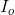, and has an intensity of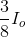after passing through both polarizes. Find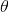?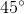Explanation:

Initially unpolarized light passing though a linear polarizer decreases in intensity by a factor of two: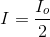Malus's Law gives the change in intensity of polarized light passing through a linear polarizer in terms of the change in angle of polarization: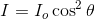Combining the two equations, we get: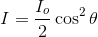Solving for: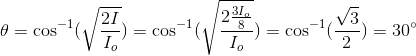### All GRE Subject Test: Physics Resources